# Chapter 22 Electric potential What is electric potential

• Slides: 25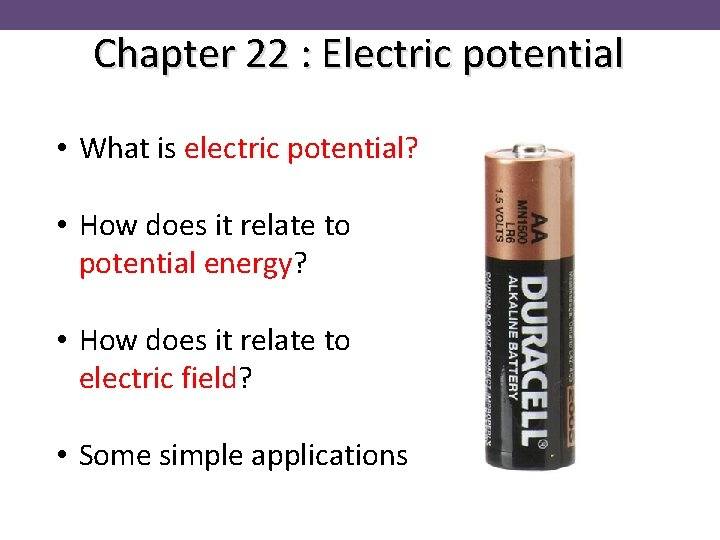Chapter 22 : Electric potential • What is electric potential? • How does it relate to potential energy? • How does it relate to electric field? • Some simple applicationsElectric potential • What does it mean when it says “ 1. 5 Volts” on the battery? • The electric potential difference between the ends is 1. 5 VoltsElectric potential 230 V 1. 5 V 100, 000 V So what is a volt?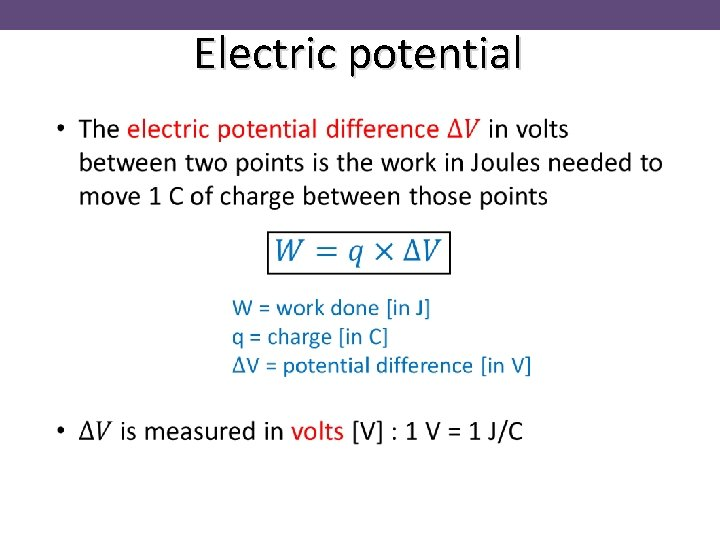Electric potentialElectric potential The 1. 5 V battery does 1. 5 J of work for every 1 C of charge flowing round the circuitPotential energy • What is thing called “potential”? • Potential energy crops up everywhere in physicsPotential energy • Potential energy U is the energy stored in a system (when work is done against a force) • e. g. force of gravity … Work = Force x DistancePotential energy • Potential energy may be released and converted into other forms (such as kinetic energy) Work is done, increasing the potential energyPotential energy • Potential energy difference is the only thing that matters – not the reference (or zero) level • For example, applying conservation of energy to a mechanics problem: Final energy = Initial energy Difference in potential energy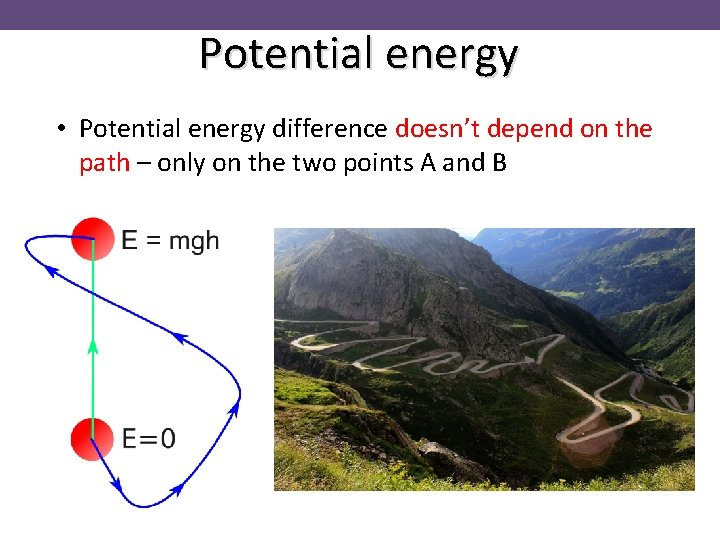Potential energy • Potential energy difference doesn’t depend on the path – only on the two points A and BPotential energy • Potential energy U is the energy stored in a system – second example • e. g. stretching a spring … Work = Force x Distance Force is varying with distance!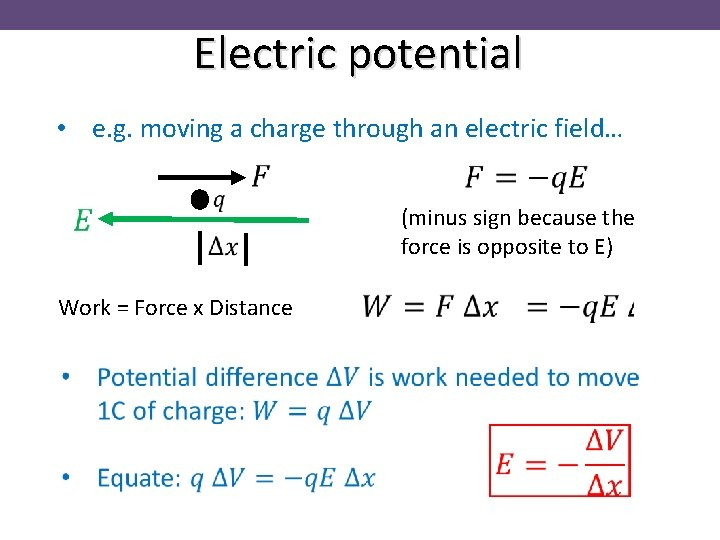Electric potential • e. g. moving a charge through an electric field… (minus sign because the force is opposite to E) Work = Force x DistanceElectric potential High V Low V • Positive charges feel a force from high to low potential • Negative charges feel a force from low to high potential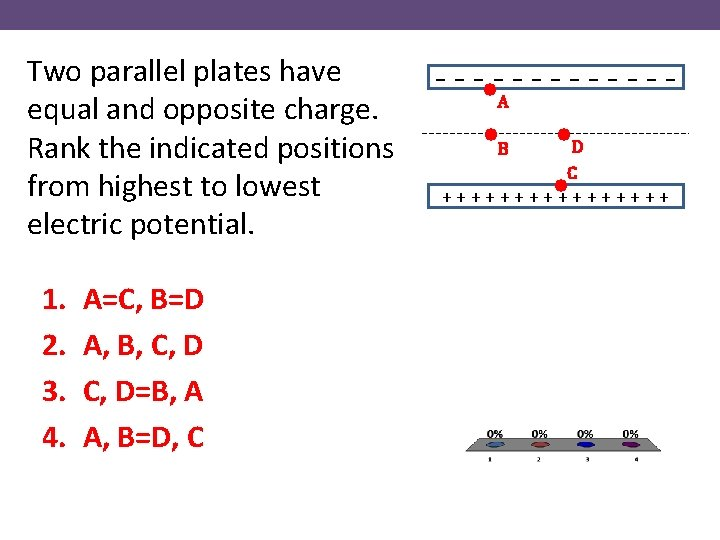Two parallel plates have equal and opposite charge. Rank the indicated positions from highest to lowest electric potential. 1. 2. 3. 4. A=C, B=D A, B, C, D=B, A A, B=D, C - - - • A • B • D C • + + + +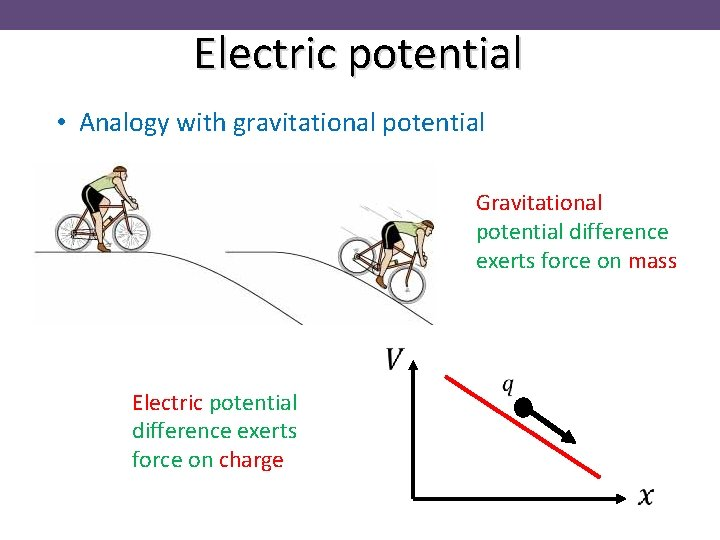Electric potential • Analogy with gravitational potential Gravitational potential difference exerts force on mass Electric potential difference exerts force on chargeElectric potential High V Low VElectric potential • Summary for two plates at potential difference V • Electric field is the potential gradient • Work W to move charge q from –ve to +ve plate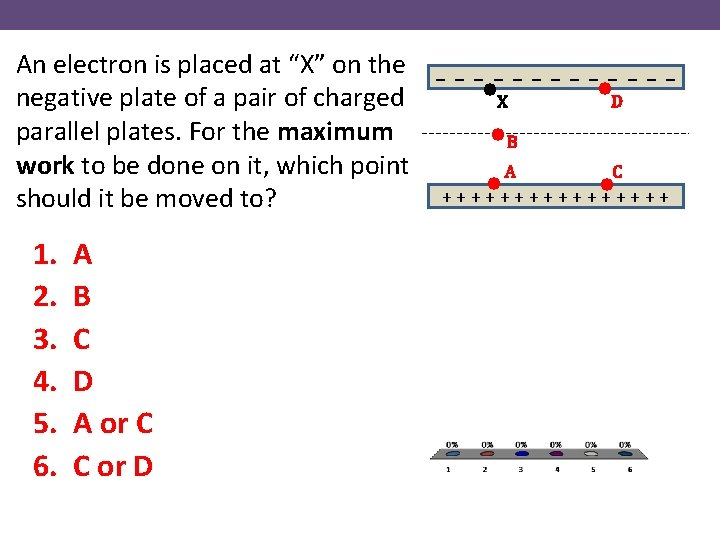An electron is placed at “X” on the negative plate of a pair of charged parallel plates. For the maximum work to be done on it, which point should it be moved to? 1. 2. 3. 4. 5. 6. A B C D A or C C or D - - - • D • X • B A C • • + + + +Electric potential • What is the electric potential near a charge +Q? Work = Force x Distance Force is varying with distance, need integral! +q + +Q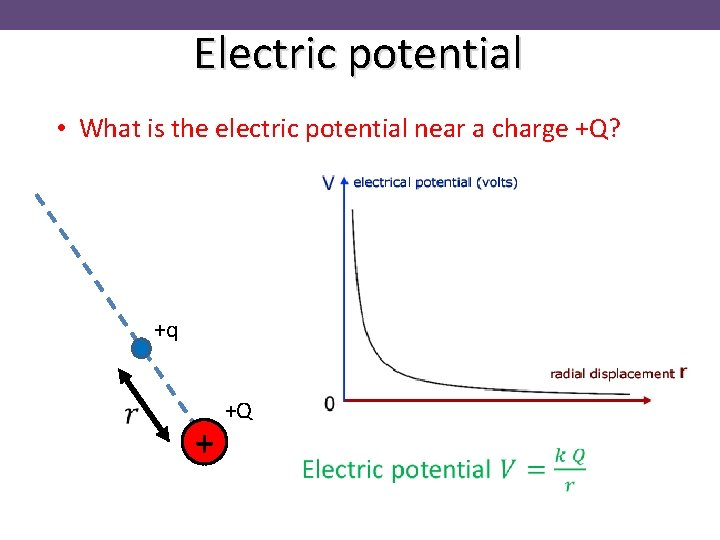Electric potential • What is the electric potential near a charge +Q? +q + +QElectric potential Exercise: a potential difference of 200 V is applied across a pair of parallel plates 0. 012 m apart. (a) calculate E and draw its direction between the plates. The electric field is the gradient in potential +ve plate -ve plate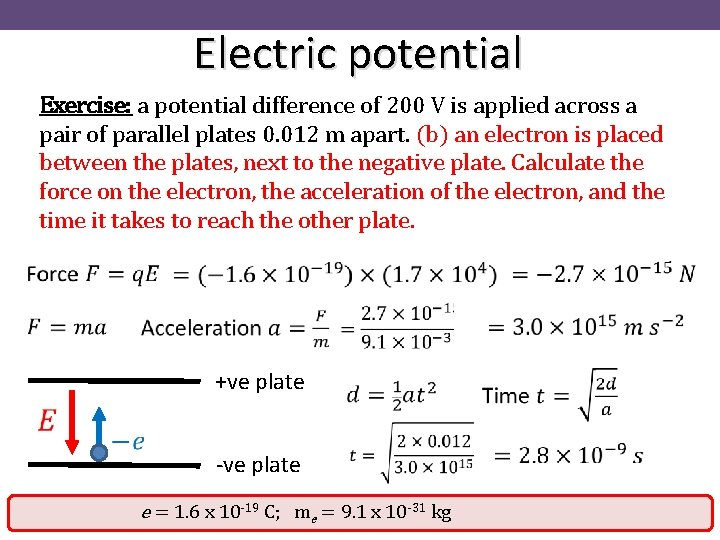Electric potential Exercise: a potential difference of 200 V is applied across a pair of parallel plates 0. 012 m apart. (b) an electron is placed between the plates, next to the negative plate. Calculate the force on the electron, the acceleration of the electron, and the time it takes to reach the other plate. +ve plate -ve plate e = 1. 6 x 10 -19 C; me = 9. 1 x 10 -31 kg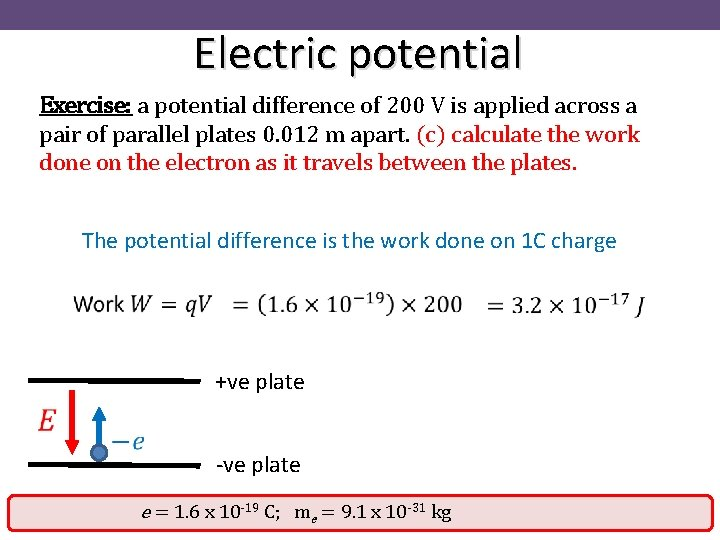Electric potential Exercise: a potential difference of 200 V is applied across a pair of parallel plates 0. 012 m apart. (c) calculate the work done on the electron as it travels between the plates. The potential difference is the work done on 1 C charge +ve plate -ve plate e = 1. 6 x 10 -19 C; me = 9. 1 x 10 -31 kg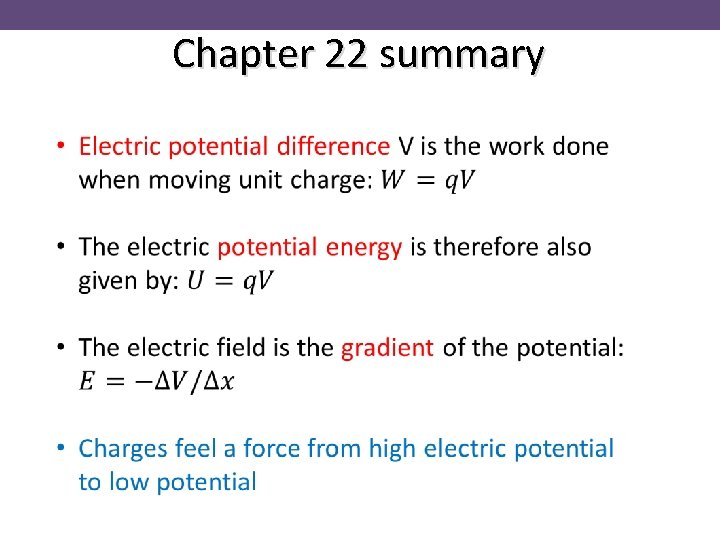Chapter 22 summary